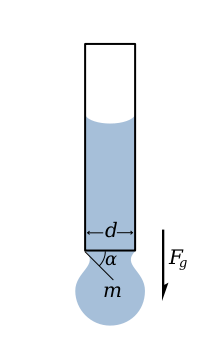homechevron_rightStudychevron_rightPhysics

# Surface tension. Weight and volume of the drop of liquid

This online calculator calculates the weight and the volume of the drop given the surface tension, diameter of the capillary tube, and density of the liquidThe picture on the left depicts a drop of liquid that is suspended from the end of a tube by surface tension. This force is proportional to the length of the boundary between the liquid and the tube and is given by

,

where - surface tension coefficient, newtons per meter, N/m

Drop is suspended as long as the vertical component of the surface tension force is equal to the gravity force, that is

The maximum applied surface tension force, when alpha equals to 90°, gives the maximum weight of a drop of liquid with a given surface tension

, thus

Having mass and density of the liquid, volume is trivial.

In reality drop detaches where "neck" is formed, slightly below the end of tube, so diameter used should be less than tube diameter. But, if high precision is not required, this is often neglected.

Also in this calculator I estimate the maximum diameter of the tube which allows drops. I assume that for drop to be formed, surface tension force should be capable to hold at least hemisphere. Thus, given density of the liquid, the formula becomes

Couple of notes on the calculator below. First, it does not calculate weight and the volume if tube diameter is more than given by equation above. Second, the default values for density of liquid and surface tension coefficient are given for water.#### Surface tension. Weight and volume of the drop of liquid

Digits after the decimal point: 3
Maximum drop weight, g

Maximum drop volume, cm3

Maximum tube diameter, m

URL copied to clipboardPLANETCALC, Surface tension. Weight and volume of the drop of liquid